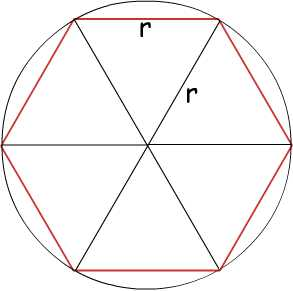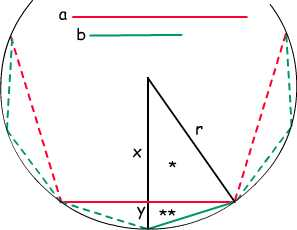# Tony’s Take March 2022

This month’s topics:

### Steven Strogatz on $\pi$ in TheNew York Times

On March 14, 2022, the Times ran “Pi Day: How One Irrational Number Made Us Modern” by Steven Strogatz. Strogatz focuses on the nature of $\pi$ as a number that can only be reached by an infinite process, so that while we can calculate more and more digits of the decimal expansion of $\pi$, the knowledge of exactly where $\pi$ sits on the number line will always be out of reach.

As Strogatz tells us, the mathematician who first made the process explicit was Archimedes (c. 287–c. 211 BCE). The Greeks had already been using the approximation of curves by polygonal lines—for example, this is how Euclid proved that the area of a circle is proportional to the square of its diameter. But determining the exact constant of proportionality does not seem to have been on his agenda. (Euclid did not explicitly consider the ratio of circumference to diameter.) It was left to Archimedes, who was an engineer as well as a mathematician, to try to nail it down.

Strogatz leads us through the process, imagining measuring the length of a circular track by walking around it and counting your steps, and multiplying that number by the length of your stride. As he explains it, each of your steps is a shortcut “in place of what really is a curved arc,” so the product you obtain will underestimate the actual length of the track. But by taking smaller and smaller steps you can get better and better estimates.

Archimedes started with an inscribed regular hexagon as a first approximation to a circle (say, of radius $r$).A regular hexagon inscribed in a circle of radius $r$.

Since a regular hexagon is made up of six equilateral triangles, the perimeter of a regular hexagon inscribed in a circle of radius $r$ is equal to $6r$. This gives a lower bound of $3$ for the ratio of circumference to diameter. As Strogath tells us, Archimedes went on to calculate the perimeters of inscribed regular polygons with 12, 24, 48 and 96 sides.

What allowed Archimedes to do these calculations is the (relatively) simple relation between the side of an inscribed regular $n$-gon and the side of the $(2n)$-gon obtained by putting a new vertex halfway between each pair of original adjacent vertices.In a circle of radius $r$, a (red} chord of length $a$ is replaced by two (green) chords of length $b$, each spanning half of the original arc. The calculation of $b$ in terms of $a$ and $r$ involves the triangles $*$ and $**$.

The red chord cuts the radius bisecting it into two segments of lengths $x$ and $y$, with $y=r-x$ . The calculation involves two applications of the Pythagorean Theorem. In triangle $*$, the theorem gives $x^2 = r^2 – (\frac{a}{2})^2$. Then in triangle $**$, it gives $b^2 = (\frac{a}{2})^2 + (r-x)^2$. The two steps, each involving extraction of a square root, give $b$ in terms of $a$ and $r$.

Archimedes repeated this calculation three more times, starting with the hexagon and $a=r$ and ending with the side of a $96$-gon. This allowed him to set $3 + \frac{10}{71}$ as a lower bound for $\pi$, while a similar calculation with circumscribed polygons yielded $3 + \frac{10}{70}$ as an upper bound. As Strogatz describes it, “The unknown value of pi is being trapped in a numerical vise, squeezed between two numbers that look almost identical, except the first has a denominator of 71 and the last has a denominator of 70.”

For Strogatz the accuracy of Archimedes’ estimates is less important than the model he gave of linear approximations to curves and the importance of iterative calculations giving sharper and sharper bounds on a number of interest. As he puts it, “Archimedes paved the way for the invention of calculus 2000 years later.”

### On math and money

The multimedia web portal Big Think ran a piece by Michael Brooks on February 2, 2022, with a nonstandard perspective on mathematical research: “More math, more money: How profit-seeking has sparked innovations in mathematics.” Brooks, the author of The Art of More: How Mathematics Created Civilization (Penguin Random House, 2022), tells us here that while we often think of math as somehow above everyday, sordid life, in fact “math and money are like Bonnie and Clyde.”

The article starts off with a reference to the “The Anatomy of a Large-Scale Hypertextual Web Search Engine” on the Stanford website, where Sergey Brin and Larry Page give among other things the mathematical definition of PageRank, an idea we know turned out to be worth a big chunk of change. But it didn’t start there. Brooks links to an article on Mesopotamian Bronze Age Mathematics by the eminent historian of science Jens Høyrup to show us how back in Ur, about 4000 years ago, King Shulgi used his knowledge of arithmetic to “implement a kingdom-wide, tamper-proof accounting system,” prevent fraud in the collection of taxes, and fill his coffers.

Moving rapidly through the centuries, Brooks takes us to When pirates studied Euclid, where Margaret Schotte (York University, Toronto) tracks in wonderful detail the merchant and privateers in 17th-century Europe as they attended special academies to learn how to use astronomy and spherical trigonometry “to deliver goods faster or, in the case of the pirates, perform better interceptions.”

Additional illuminating examples cover algebra, calculus, and statistics. The moral of the story: “No one should be aiming to become a singer or a sports star. Math is a much more reliable road to riches.”

### “We’re all still living in Euclid’s world”An illustration like this one, a dodecahedron superimposed on the picture of a galaxy, was used to illustrate Wilczek’s article. Here the galaxy (NGC 4414) is from NASA.

“The geometry of ancient Greece has stood for more than two millennia, even after relativity and quantum mechanics” is the rest of the title of a short article by Frank Wilczek. The Nobel prize-winning physicist is a regular contributor to The Wall Street Journal, where the piece ran on February 4, 2022.

Euclid’s Elements was written around 300 BCE. It built geometry up from some more or less obvious definitions and from a few “axioms,” supposed to be self-evident assumptions about how our geometrical world works. As Wilczek reminds us, the Elements, beyond training generations of students “not only in the science of space and measurement but in the art of clear thinking and logical deduction,” provided the framework for Newton’s physics and later for Maxwell’s theory of electromagnetism.

But then something happened. Euclid’s Axiom 5, the “parallel postulate” (equivalent to the statement that the angles in a triangle add up to $180^{\circ}$), felt different from the others; in the 19th century, mathematicians realized that it could be modified in two different ways and still give a coherent, uniform geometry. In one direction the sum of the angles in a triangle is always more than $180^{\circ}$. This corresponds to the situation on the surface of the Earth, where navigators build triangles out of segments of great circles, the spherical equivalent of straight lines. In the other direction, the sum is always less than $180^{\circ}$. This second geometry was discovered by Gauss (who didn’t publish it) and independently by Lobachevsky and Bolyai. As opposed to the curvature of a spherical surface, which we call positive, these “hyperbolic” surfaces have negative curvature, like the surface of a saddle, curling up in one direction and down in the other.

Not many years later Bernhard Riemann came up with the concept of what we now call a Riemannian manifold, where the curvature can vary from point to point. The equivalent of a straight line on such a surface (a path giving the shortest distance between two of its points) could be quite far from straight. Wilczek gives an example from sports: “an Alpine skier racing down a bumpy mountain will keep doing her best to go straight down, but over the course she will trace a curve.” Riemann himself was interested in the geometry of the three-dimensional space we live in, and his manifolds can have 2, 3, 4, … any number of dimensions.

Wilczek leads us through the next development. It started with Einstein’s special theory of relativity, developed to explain the non-intuitive fact that your measurement of the speed of light does not depend on how fast you are moving. The mixture of space and time coordinates that Einstein required was given a geometric formulation by Minkowski who stated in 1908, as Wilczek quotes him, “Henceforth space by itself, and time by itself, are doomed to fade away into mere shadows, and only a kind of union of the two will preserve an independent reality.” Einstein used this formalism for his next step, the general theory of relativity, where space-time has curvature, analogous to the Riemannian concept we just saw, and intimately related to gravity. (The measurement, made during the 1919 total solar eclipse, of how much light rays—by definition, straight lines in space-time—would curve when passing close to the sun was experimental proof of the theory.)

This sounds pretty far removed from similar triangles and the Pythagorean theorem, but Wilczek assures us that “Einstein’s framework is still recognizably Euclidean, extended and adapted to bring in time and large-scale curvature.” In fact, he considers this “the most striking example of what Eugene Wigner called ‘the unreasonable effectiveness of mathematics in the natural sciences.'”

### Symmetry in biology, why?A schematic representation of the light-harvesting complex in the membrane of the bacterium Rhodopseudomoas acidophila. Image generated by Iain Johnston from data in Papiz et al. (2003) J Mol Bio 326 1523-1538, used with permission.

Kate Golembiewski wrote “Life’s Preference for Symmetry Is Like ‘A New Law of Nature,'” including the above image, for The New York Times. It appeared in the Trilobites section online on March 24, 2022, and a shorter version appeared in print on the 29th. The article reports on “Symmetry and simplicity spontaneously emerge from the algorithmic nature of evolution” by Iain Johnston, Kamaludin Dingle, and collaborators, published in PNAS on March 15.

As we know, symmetry is everywhere in nature: Starfish have 5-fold symmetry, flowers have bilateral or rotational symmetry (of various orders), virus capsids have icosahedral symmetry, our left hands are almost exact mirror-images of our right. The question is why. “Biologists aren’t sure,” Golembiewski tells us, “—there’s no reason based in natural selection for symmetry’s prevalence in such varied forms of life and their building blocks.”

It turns out that the study of algorithms can point to an answer. As Golembiewski relates, the authors of the PNAS paper concluded that symmetry is evolutionarily favored because it makes the storage and replication of genetic data easier and more reliable. “Dr. Johnston […] likens it to telling someone how to tile a floor: It’s easier to give instructions to lay down repeating rows of identical square tiles than explain how to make a complex mosaic.” This is an explanation distinct from natural selection! Chico Camargo, another of the authors, told her: “It’s like we found a new law of nature. […] This is beautiful, because it changes how you see the world.”